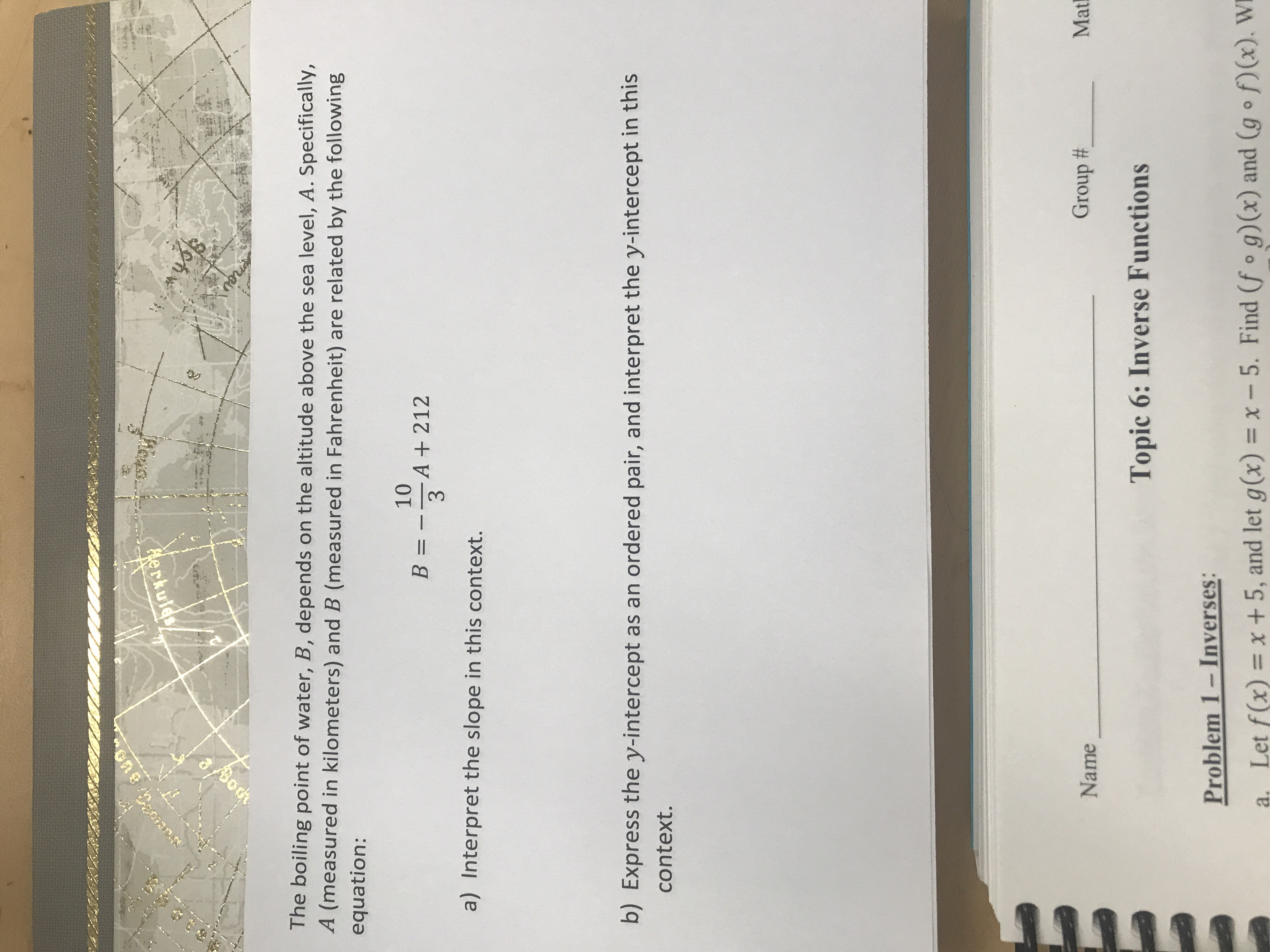# 2#3The boiling point of water, B, depends on the altitude above the sea level, A. Specifically,A (measured in kilometers) and B (measured in Fahrenheit) are related by the followingequation:10A +2123B =a) Interpret the slope in this context.b) Express the y-intercept as an ordered pair, and interpret the y-intercept in thiscontext.Group #MatNameTopic 6: Inverse FunctionsProblem 1-Inverses:Let f(x) = x + 5, and let g(x) = x- 5. Find (fo g) (x) and (g f)(x). W

Question
10 viewshelp_outlineImage Transcriptionclose2#3 The boiling point of water, B, depends on the altitude above the sea level, A. Specifically, A (measured in kilometers) and B (measured in Fahrenheit) are related by the following equation: 10 A +212 3 B = a) Interpret the slope in this context. b) Express the y-intercept as an ordered pair, and interpret the y-intercept in this context. Group # Mat Name Topic 6: Inverse Functions Problem 1-Inverses: Let f(x) = x + 5, and let g(x) = x- 5. Find (fo g) (x) and (g f)(x). W fullscreen
check_circle

Step 1

Part (a)

Compare the given equation: B = (-10/3)A + 212 with the standard equation of a striaght line: y = mx + c

The slope = m = - 10/3 = dy/dx = dB / dA

Hence, the slope in this context mens the rate of change of B with resepct to A. The negative sign indicates t...

### Want to see the full answer?

See Solution

#### Want to see this answer and more?

Solutions are written by subject experts who are available 24/7. Questions are typically answered within 1 hour.*

See Solution
*Response times may vary by subject and question.
Tagged in

### Functions